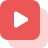Home » About GATE » GATE study materials » Chemical Engineering » NEWTONIAN AND NON-NEWTONIAN FLUIDS

# NEWTONIAN AND NON-NEWTONIAN FLUIDS

Newton gave Newton's law of viscosity, the fluid which follows Newton’s law of viscosity is called Newtonian fluid and which does not follow Newton’s law of viscosity is called non-Newtonian fluids. In today's life you witnessed some and now also you witness some Newtonian fluids and non-Newtonian fluids. Fluids like motor oil, water etc. are Newtonian fluids and fluids like toothpaste, butter etc. are non-Newtonian fluids.

Sir Isaac Newton (25 Dec 1642- 20 Mar 1727) gave Newton’s law of viscosity; the fluid which follows Newton’s law of viscosity is called Newtonian fluid and which does not follow Newton’s law of viscosity is called non-Newtonian fluids. Any fluid can be classified as 2 types.

1. Newtonian fluids
2. Non-Newtonian fluids

### NEWTONIAN FLUIDS:

Newton’s law of viscosity states that “viscosity is not dependent on shear; that is viscosity is independent whatever the shear state is”. Fluids that follow Newton’s law are called Newtonian fluid. A Newtonian Fluid will take the form of its container in which it is kept. In this law, Newton gave a linear relationship between shear stress and shear rate.

τ   =     η     ×    ⅈ

shear stress=viscosity ×shear strain rate

This formula can also be written as:

τ  =       ×     du/dy

τ  =shear stress

= dynamic viscosity

When viscosity is constant, whatever the shear rate is, then the fluid is called Newtonian fluid.

### CHARACTERISTICS OF NEWTONIAN FLUIDS:

Some important characteristics of Newtonian Fluid is:.

• Newtonian fluids are non-compressible.
• Newtonian fluids are unreactive.
• Newtonian fluids are isotropic.
• At fixed temperature, Newtonian fluids viscosity doesn’t change.
• Newtonian fluid follows Newton’s law of viscosity.

### CLASSIFICATION OF NEWTONIAN FLUID:

Newtonian fluids are classified depending on the relationship between the shear stress applied to the flow and the speed of deformation of stress. Fluids, in which shear stress is directly proportional to the deformation rate, are called Newtonian fluids.

### NON-NEWTONIAN FLUIDS:

A Non-Newtonian fluid is a fluid that when it comes under stress, its flow viscosity, and flow behavior changes. When force is applied to such fluids, then stress can cause them to get thicker so that it will act like a solid, or in some cases it acts exactly opposite behavior and may increase in their running behavior. When stress is removed and leaves the fluid still, it will return to its earlier state.

Say you would like to get some tomato sauce out of the bottle. You find there is some in there, but once you turn the bottle the wrong way up, nothing comes out. So, what do you do? You shake or hit the bottle. This causes the tomato sauce to become more liquid and you’ll be able to easily squirt some out. Hence, sauce’s viscosity decreases and applied stress makes it runnier.

Oobleck is a mixture of cornflour and water. This liquid changes its characteristics when you apply stress to that liquid; that is, it acquires solidity when shear stress is applied to it. You’ll be able to hit a bowlful with a hammer, and rather than splashing everywhere, the particles lock together. You’ll be able to roll it into a solid ball in your hand, but if you stop moving it, it reverts to liquid and comes out through your fingers. In this case, the oobleck’s internal resistance to flow, that means its viscosity increases due to the applied stress.

Non-Newtonian fluids are classified into four types depending upon:

• Viscosity or Measuring fluids internal resistance to flow.
• Change in fluid characteristics with respect to time.
• Change in fluid characteristics with respect to magnitude of applied shear rate.

The four types of non-Newtonian fluids examples are:

• Thixotropic fluids: these are the fluids, when shear stress is applied, its characteristics decrease over time. Example: Continuous stirring of solid honey changes its characteristics and makes it liquid honey.
• Rheopectic fluids: these are the fluids, when stress is applied, its characteristics increase over time. Example: continuous stirring of cream changes its characteristics and makes it thicken.
• Pseudoplastic fluids: These are the shear thinning materials. The term shear thinning is referred to when an increase in fluid shear rate causes the decrease in fluid viscosity. Shear thinning fluids are also known as thixotropic.

Dilatant Fluids: These are the shear thickening materials. The term shear thickening is referred to when an increase in a fluid shear rate causes the increase in fluid viscosity. Shear thickening materials are also known as dilatant.

### CONCLUSION:

Newtonian Fluids and Non-Newtonian Fluids knowledge should be important so that fluid mechanics and fluids industrial uses should be easier. These Newtonian fluids examples and non-Newtonian fluids examples that are provided by this article are used in daily life. Any fluid can come under these two types of classification.Get answers to the most common queries related to the GATE Examination Preparation.

## How does stability work in a fluid static?

Ans: An object remains stable when “an angular displacement moves the margin of action of an upwa...Read full

## What are the factors for a fluid to be considered static?

Ans: Static fluid refers to the fact that there is no motion in the fluid, which has equal pressure...Read full

## Which forces mainly deal in static fluids?

Ans: Due to the pressure changes, the friction between the molecules has been seen, these differenc...Read full

## What are the applications of fluid statics?

Ans: It helps to measure the pressure with hydrostatics, floating or submerged ships or submarines,...Read full